Home > CC4 > Chapter A > Lesson A.1.4 > ProblemA-42

A-42.

Evaluate each equation below. Homework Help ✎

1. For f(x) = 2 + 3x, what is the value of f(4)?

2. For a = 4 − 5c when c = −, what is the value of a?

3. For n = 3d 2 − 1 when d = −5 , what is the value of n?

4. For h(x) = −4(x − 2), what is the value of h(−1)?

5. For 3 + k = t when t = 14, what is the value of k?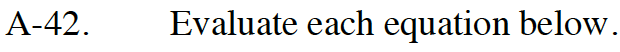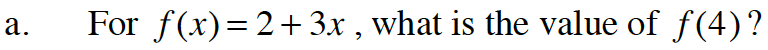Rewrite the expression, substituting in the known variable.

y = 2 + 3(4)
y = 2 + 12

y = 14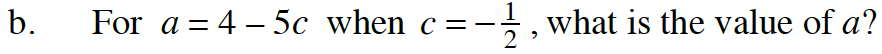See part (a) for a strategy to evaluate this expression.
Remember to pay attention to negative signs.

a = 6.5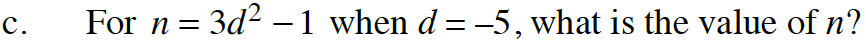Rewrite the expression, substituting in the known variable.

n = 3(−5)² − 1
n = 3(25) − 1
n = 75 − 1

n = 74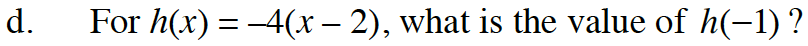Refer to parts (a) and (c).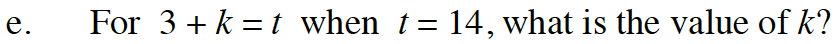Rewrite the problem, substituting in the known variable and then rearrange the problem to make it equal to the unknown variable.